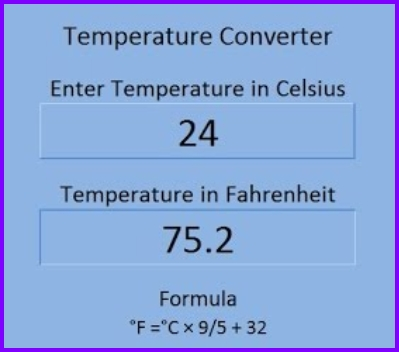# Temperature Conversion Template

Exceltemplates.org – Many students confused about temperature conversion because they do not understand the differences between each temperature scale. They do not know the difference between Celsius, Kelvin and Fahrenheit. Actually, it is an easy discussion. There are some effective methods can be used to learn about it, including this temperature conversion worksheet.This excel template was created several years ago. This template was created by interpreting the temperature conversion formula into the correct excel function. Actually, the excel function is already has it. The conversion function there named “Convert”. This function can be used by anyone not just for converting temperature but also for converting other conversions, such as distance, time, weight and many more. Excel provides conversion for temperature between Fahrenheit, Celsius and also Kelvin. The implementation can be seen in the excel spreadsheet.

Before entering the worksheet, it is better for you to understand the definition of each temperature scale.

• Fahrenheit

Fahrenheit scale is the scale we usually heard on the weather report on the news. This scale maybe is the most familiar for us, especially if we live in United States. Most temperatures sold in the United States are using Fahrenheit. However, in Canada and many other countries, the weather reports are using Celsius scale.

• Celsius scale

This is the scale that is commonly used in scientific works. Most thermometers that are used in laboratory are using this scale. This scale is also called the Centigrade scale because it was specially designed and created in such a way that there are 100 units or degrees between the boiling point and freezing point of water. Negative temperatures are something common in the Celsius scale.

• Kelvin scale
Check this template >  College Budget Calculator

The International System of Measurements uses this scale for measuring temperature. Kelvin scale makes more sense in light of the way that temperature is described. This temperature scale is created based on the concept of absolute zero, which a temperature theory describes that molecules would have only zero kinetic energy. The absolute zero is about -273.15 degrees Celsius and it is set at zero on the Kelvin scale. It means, there is not temperature lower than zero Kelvin or there are no negative temperatures on this scale.

The conversion formula between each scale is:

• Fahrenheit to Celsius » C = (F – 32) X 5/9
• Celsius to Fahrenheit » F = (CX9/5) + 32
• Celsius to Kelvin » K = C + 273
• Kelvin to Celsius » C = K – 273

For details about the temperature conversion, you can download and print the worksheet in this page. This worksheet was created in excel template, so you can easily print it based on your needs. You can use any paper size you want.

You must know that understanding about temperature conversion is something important, especially if you are students. Mathematics, chemistry, physics and engineering are some disciplines that require you to understand about temperature conversion. With this worksheet, you can learn about the conversion without have to open too many books or access to many webpages.